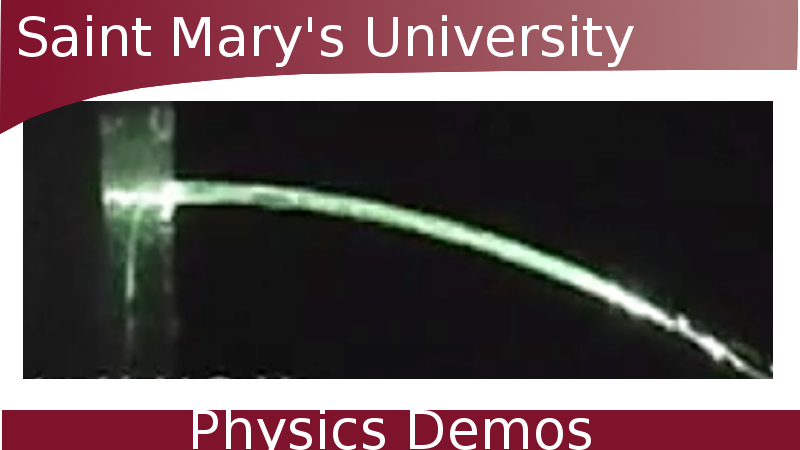#### refraction in water.

Watch Video:

Other Demos of Interest:Water Acting as a Fibre-Optic Bending Water with Static Electricity Refraction of Waves

Teachable Topics:
• Refraction
• Snell's Law

Theory:

Refraction is what happens when light bends while travelling from one medium into another. The amount the light bends depends on a number of factors, such as the angle of the incoming (incident) light beam to the new medium, and the index of refraction of the media involved.

The index of refraction, n, is a ratio of the speed of light in a vacuum and the speed of light in the medium. It is given by:

#### n = c / vmedium

where c is the speed of light in a vacuum and vmedium is the speed of light in the medium. As such, the index of refraction of a vacuum is 1 while the index of refraction of air is very close at 1.0003. The index of refraction for water is 1.33.

When a ray of light is refracted while travelling from one medium (with an index of refraction of n1) into another (with an index of refraction of n2), the following relation is used:

#### n1sin(θ1) = n2sin(θ2)

where θ1 is the angle of incidence and θ2 is the angle of refraction. This relationship is known as Snell's Law, named after Dutch scientist Willebrord Snell.

In this demo, the light rays from the quarter are moving from water into the air. Because the index of refraction for water is greater than air, the angle of refraction will be greater than the angle of incidence. This means the light rays bend towards us, making the quarter visible. This is the same effect that causes straws to look broken or bent in a glass of water.

Apparatus:

• Mug
• A coin (we used a quarter here)
• Tape
• Water

Procedure:

• Fasten the quarter to the bottom of the mug with the tape.
• Position yourself at an angle where you can no longer see the coin.
• Add water to the mug until the quarter becomes visible again.